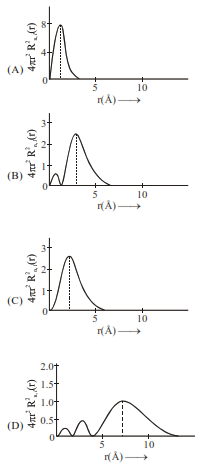# The plots of radial distribution`
Question:

The plots of radial distribution functions for various orbitals of hydrogen atom against ' $r$ ' are given below:The correct plot for $3 \mathrm{~s}$ orbital is:

1. (B)

2. (A)

3. (D)

4. (C)

Correct Option: , 3

Solution:

Number of radial nodes $=\mathrm{n}-\ell-1$

$=3-0-1=2$

Therefor corresponding graph is (D)